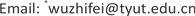1大运汽车股份有限公司，山西 运城

2太原理工大学，山西 太原1. 引言

2. 四分之一悬架模型的建立

m z x ¨ z + c ( x ˙ z − x ˙ f ) + k z ( x z − x f ) − u = 0 m f x ¨ f − c ( x ˙ z − x ˙ f ) − k z ( x z − x f ) + k f ( x f − q ) + u = 0 (1)

q ˙ ( t ) = − a v q ( t ) + w ( t ) (2)

3. 模糊控制器的设计

e = [ − 6 , 6 ] e c = [ − 6 , 6 ] u = [ − 6 , 6 ] (3)

K e = = 40 K e c = m / e c = 4 K u = x / u = 115 (4)

Fuzzy rule control tabl
EcE
NBNMNSZOPSPMPB
NBPBPBPMPMPSZOZO
NMPBPBPMPSPSZONS
NSPMPMPMPSPSZONS
ZOPMPMPSZONSNSNM
PSPSPSZONSNSNMNM
PMPSZONSNSNMNMNB
PNZOZONMNMNMNBNB

4. 仿真分析

Comparison of smoothness evaluation indicator

5. 结论

1) 车辆行驶路面的不平度是随机性的，本文以随机白噪声作为系统的输入，来模拟随机路面，和实际路况相符。

2) 本文采用模糊控制的策略建立了四分之一车辆半主动悬架模型，通过本文提出的模糊控制策略控制主动控制力的实时输出，有效地改善了电动车悬架系统的的平顺性和操纵稳定性。验证了具有模糊控制器的半主动悬架对电动车悬架动态特性的改善有良好的效果。同时，模糊控制系统搭建简便，使得半主动悬架系统的建立具有实际意义。